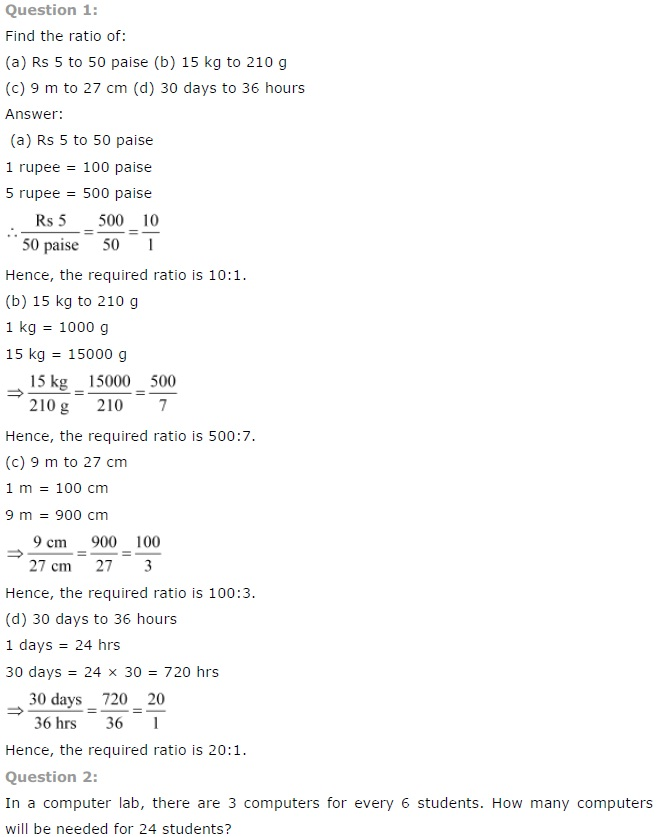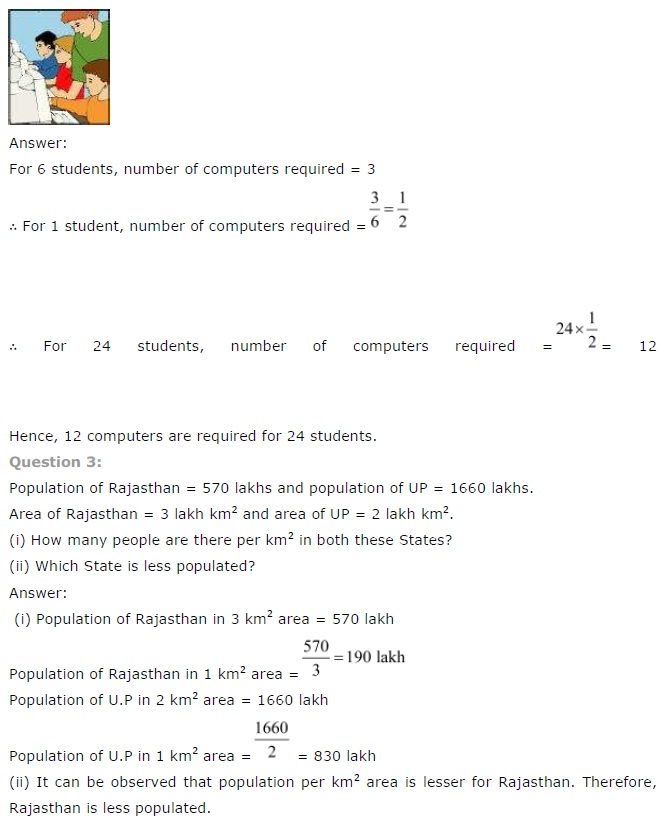# NCERT Solutions for Class 7 Maths Chapter 8 Comparing Quantities

NCERT Solutions for Class 7 Maths Chapter 8 Comparing Quantities

### NCERT Solutions for Class 7 Maths Chapter 8 Comparing Quantities Ex 8.1

NCERT Solutions for Class 7 Maths Chapter 8 Comparing Quantities Exercise 8.1
Ex 8.1 Class 7 Maths Question 1.
Find the ratio of:
(a) ₹ 5 to 50 paise
(b) 15 kg to 210 g
(c) 9 m to 27 cm
(d) 30 days to 36 hours
Solution:
(a) ₹ 5 to 50 paise
Converting the given quantities into same units, we have
₹ 5 = 5 × 100 = 500 paise
∴ ₹ 5 : 50 paise
= 500 paise : 50 paise [∵ ₹ 1 = 100 paise]
= 10 : 1
So, required ratio is 10 : 1.

(b) 15 kg to 210 g
Converting the given quantities into same units, we have
15 kg = 15 × 1000
= 15000 g [∵ 1 kg = 1000 g]
∴ 15 kg : 210 g = 15000 g : 210 g
= 1500 : 21
= 500 : 7
So, the required ratio is 500 : 7.

(c) 9 m to 27 cm
Converting the given quantities into same units, we have
9 m = 9 × 100 = 900 cm
∴ 9m: 27 cm = 900 cm : 27 cm [∵ 1 m = 100 cm]
= 100 : 3
So, the required ratio is 100 : 3.

(d) 30 days to 36 hours
Converting the given quantities into same
units, we have
30 days = 30 × 24 hours [ ∵ 1 day = 24 hours]
= 720 hours
∴ 30 days : 36 hours
= 720 hours : 36 hours = 20:1
So, the required ratio is 20 : 1.

Ex 8.1 Class 7 Maths Question 2.
In a computer lab, there are 3 computers for every 6 students. How many computers will be needed for 24 students?
Solution:Ex 8.1 Class 7 Maths Question 3.
Population of Rajasthan = 570 lakhs and population of UP = 1660 lakhs.
Area of Rajasthan = 3 lakh km2 and area of UP = 2 lakh km2.
(i) How many people are there per km2 in both these States?
(ii) Which State is less populated?
Solution:
Given:
Population of Rajasthan = 570 lakhs
Population of UP = 1660 lakhs
Area of Rajasthan = 3 lakh km2
Area of UP = 2 lakh km2## SabDekho

The Complete Educational Website